# KR101687217B1 - Robust face recognition pattern classifying method using interval type-2 rbf neural networks based on cencus transform method and system for executing the same - Google Patents

Robust face recognition pattern classifying method using interval type-2 rbf neural networks based on cencus transform method and system for executing the same Download PDF

## Info

Publication number
KR101687217B1
KR101687217B1 KR1020150169441A KR20150169441A KR101687217B1 KR 101687217 B1 KR101687217 B1 KR 101687217B1 KR 1020150169441 A KR1020150169441 A KR 1020150169441A KR 20150169441 A KR20150169441 A KR 20150169441A KR 101687217 B1 KR101687217 B1 KR 101687217B1
Authority
KR
South Korea
Prior art keywords
neural network
algorithm
rbf neural
interval type
type
Prior art date
Application number
KR1020150169441A
Other languages
Korean (ko)
Inventor
오성권
Original Assignee
수원대학교산학협력단
위아코퍼레이션 주식회사
Priority date (The priority date is an assumption and is not a legal conclusion. Google has not performed a legal analysis and makes no representation as to the accuracy of the date listed.)
Filing date
Publication date
Application filed by 수원대학교산학협력단, 위아코퍼레이션 주식회사 filed Critical 수원대학교산학협력단
Priority to KR1020150169441A priority Critical patent/KR101687217B1/en
Application granted granted Critical
Publication of KR101687217B1 publication Critical patent/KR101687217B1/en

## Links

• 230000001537 neural Effects 0.000 title claims abstract description 64
• 238000007781 pre-processing Methods 0.000 claims abstract description 11
• 238000004458 analytical method Methods 0.000 claims abstract description 10
• 238000000034 method Methods 0.000 claims description 38
• 230000001131 transforming Effects 0.000 claims description 17
• 230000004913 activation Effects 0.000 claims description 11
• 238000002939 conjugate gradient method Methods 0.000 claims description 9
• 239000011324 bead Substances 0.000 claims description 2
• 238000005516 engineering process Methods 0.000 abstract description 5
• 239000011159 matrix material Substances 0.000 description 19
• 238000005286 illumination Methods 0.000 description 14
• 238000010586 diagram Methods 0.000 description 8
• 230000001815 facial Effects 0.000 description 7
• 238000005457 optimization Methods 0.000 description 6
• 241000256844 Apis mellifera Species 0.000 description 5
• 238000010200 validation analysis Methods 0.000 description 5
• 238000002474 experimental method Methods 0.000 description 4
• 238000000605 extraction Methods 0.000 description 3
• 238000000926 separation method Methods 0.000 description 3
• 241000257303 Hymenoptera Species 0.000 description 2
• 230000001174 ascending Effects 0.000 description 2
• 230000003542 behavioural Effects 0.000 description 2
• 238000002247 constant time method Methods 0.000 description 2
• 238000004904 shortening Methods 0.000 description 2
• 241000704611 Fig cryptic virus Species 0.000 description 1
• 238000007796 conventional method Methods 0.000 description 1
• 230000000875 corresponding Effects 0.000 description 1
• 238000002790 cross-validation Methods 0.000 description 1
• 230000003247 decreasing Effects 0.000 description 1
• 230000000694 effects Effects 0.000 description 1
• 238000011156 evaluation Methods 0.000 description 1
• 230000004807 localization Effects 0.000 description 1
• 238000003062 neural network model Methods 0.000 description 1
• 238000003909 pattern recognition Methods 0.000 description 1
• 238000007619 statistical method Methods 0.000 description 1

## Images

•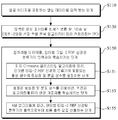•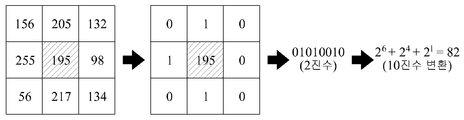••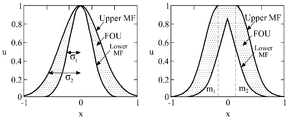•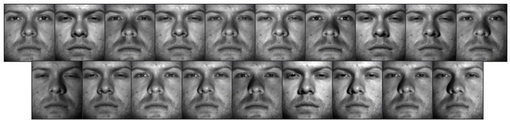••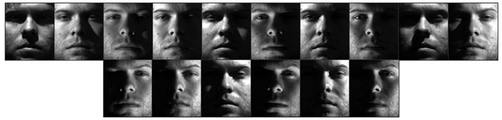•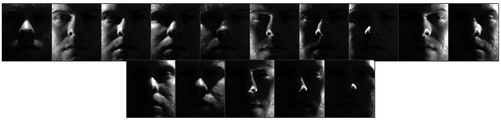•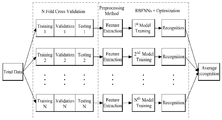•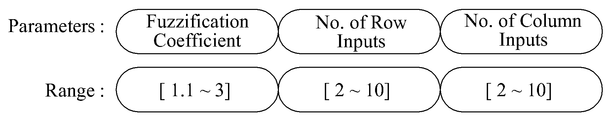•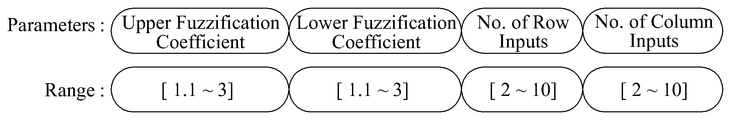## Classifications

• GPHYSICS
• G06COMPUTING; CALCULATING; COUNTING
• G06KRECOGNITION OF DATA; PRESENTATION OF DATA; RECORD CARRIERS; HANDLING RECORD CARRIERS
• G06K9/00Methods or arrangements for reading or recognising printed or written characters or for recognising patterns, e.g. fingerprints
• G06K9/00221Acquiring or recognising human faces, facial parts, facial sketches, facial expressions
• G06K9/00288Classification, e.g. identification
• GPHYSICS
• G06COMPUTING; CALCULATING; COUNTING
• G06KRECOGNITION OF DATA; PRESENTATION OF DATA; RECORD CARRIERS; HANDLING RECORD CARRIERS
• G06K9/00Methods or arrangements for reading or recognising printed or written characters or for recognising patterns, e.g. fingerprints
• G06K9/00221Acquiring or recognising human faces, facial parts, facial sketches, facial expressions
• G06K9/00228Detection; Localisation; Normalisation
• GPHYSICS
• G06COMPUTING; CALCULATING; COUNTING
• G06KRECOGNITION OF DATA; PRESENTATION OF DATA; RECORD CARRIERS; HANDLING RECORD CARRIERS
• G06K9/00Methods or arrangements for reading or recognising printed or written characters or for recognising patterns, e.g. fingerprints
• G06K9/36Image preprocessing, i.e. processing the image information without deciding about the identity of the image
• GPHYSICS
• G06COMPUTING; CALCULATING; COUNTING
• G06KRECOGNITION OF DATA; PRESENTATION OF DATA; RECORD CARRIERS; HANDLING RECORD CARRIERS
• G06K9/00Methods or arrangements for reading or recognising printed or written characters or for recognising patterns, e.g. fingerprints
• G06K9/62Methods or arrangements for recognition using electronic means
• G06K9/6267Classification techniques
• G06K9/6268Classification techniques relating to the classification paradigm, e.g. parametric or non-parametric approaches
• G06K9/627Classification techniques relating to the classification paradigm, e.g. parametric or non-parametric approaches based on distances between the pattern to be recognised and training or reference patterns

## Abstract

The present invention relates to a robust face recognition pattern classifying method using an interval type-2 radial basis function (RBF) neural network based CT technology and a system for executing the same. The method comprises the following steps of: receiving image data including a face image; preprocessing the received image data in accordance with a census transform algorithm and a two-dimensional two-directional linear discriminant analysis algorithm; and inputting the preprocessed data to an interval type-2 RBF neural network classifier to learn. Therefore, the method can improve face recognition performance.

## Description

TECHNICAL FIELD  The present invention relates to a method of classifying face recognition patterns using a CT method based on an interval type-2 RBF neural network, and a system for implementing the method. }
The present invention relates to a face recognition pattern classification method, and more particularly, to a robust face recognition pattern classification method using an interval type-2 RBF neural network based CT technique and a system for implementing the same.
Biometrics refers to the technique of identifying an individual by measuring an individual's physical or behavioral characteristics with an automated device. With biometrics, identification passwords do not need to be memorized separately, and they are becoming popular in real life because they have to be in person. Face recognition during biometrics has the advantage of making the user feel less uncomfortable by non-contact type.
In this regard, the technique of automatically recognizing faces from still images or moving images is actively connected in various fields such as image processing, pattern recognition, computer vision and neural network, and has numerous commercial and legal applications. These applications range from the use of limited forms of still images such as passports, credit cards, resident registration cards, driver's licenses, and face pictures of criminals to real-time recognition such as video surveillance.
Face image recognition technology can be generally defined as checking whether a still image or a moving image of a given background exists in a database in which one or more persons are present in the input image. Incidental information such as race, age, sex, etc. may also be used to narrow the search area.
This facial image recognition technology consists of separation of face region, extraction of facial features, and classification process. In addition to recognition using front face images, facial recognition technology using side face images can be considered as another method. In this method, the distance between reference points of side faces is typically used as a feature. The facial recognition method using the side face image has not been studied so far due to the constraint applied at the time of photographing. However, since it is more accurate than the method using the front face image, it is mainly used for problems .
The face recognition method using the still image has several advantages and disadvantages. For example, when dealing with the problem of finding the criminal among the photographs of the sinner, the separation of the face may be made easier due to various constraints However, it is difficult to separate faces in images with complex backgrounds like airports. On the other hand, in the video obtained from the camera, it is easier to separate the face by using human motion as a clue. Research on the problem of separating the background has been actively conducted, and researches for separating not only a face but also a moving object are actively under way.
The present invention has been proposed in order to overcome the above-mentioned problems of the conventional methods. The present invention proposes a method in which input data including a face image is preprocessed to be robust against illumination change through a census transformation algorithm, The initial parameters are set to the connection weights obtained from the type-1, and the fuzzy C- means clustering, the number of learning times of the backpropagation algorithm process is reduced and the computation time is reduced by optimizing the number of input of the fuzzy coefficients and the number of sides / columns, thereby improving the face recognition performance according to the optimized number of inputs. Robust Face Recognition Pattern Classification Method Using RBF Neural Network Based CT Technique and System for Implementing It To provide it for that purpose.
According to another aspect of the present invention, there is provided a method for classifying a face recognition pattern using a CT method based on an interval type-2 RBF neural network,
(1) receiving image data including a face image;
(2) preprocessing the input image data according to a census transformation algorithm and a two-dimensional-two-way linear discriminant analysis algorithm; And
(3) inputting the preprocessed data to an interval type-2 RBF (radial basis function) neural network classifier,
The step (3)
(3-1) setting a center point and a distribution constant of an activation function included in the interval type-2 RBF neural network classifier according to a fuzzy C-means clustering algorithm; And
(3-2) learning the connection weight according to the back propagation algorithm using the set center point and the distribution constant.
Preferably, the step (3)
(3-a) optimizing the fuzzy coefficient of the interval type-2 RBF neural network classifier using an artificial bead clustering algorithm.
Preferably, the step (3)
(3-3) calculating a final output value from the output of the interval type-2 RBF neural network classifier according to a KM (Karnik and Mendel) algorithm.
More preferably, in the step (4)
And to average the outputs of the interval type-2 RBF neural network classifiers to calculate the final output value.
Preferably,
The activity function of the interval type-2 RBF neural network classifier used in step (3) may be configured to include a type-2 fuzzy set of Gaussian type.
Preferably, in the step (3-2)
The back propagation algorithm may be configured to use a conjugate gradient method.
More preferably,
The direction vector used to express the interval value of the parameter coefficient or connection weight of the next generation is expressed using the product of the direction vector of the previous generation and the coefficient beta (t), and the coefficient beta (t) Is expressed by the following equation by the vector G (t-1) and the gradient vector G (t) of the current generation,
If the value of the above equation exceeds 1, the coefficient? (T) may be configured to be set to one.
According to the robust face recognition pattern classification method using the interval type-2 RBF neural network-based CT technique proposed in the present invention and the system for executing the same, input data including a face image is robust to illumination change through a census transformation algorithm And the characteristics of the transverse and the column are extracted through the two-dimensional and two-directional linear discriminant analysis, and input to an interval type-2 RBF neural network in which a type-2 fuzzy set is combined, And the fuzzy coefficient of the fuzzy C-means clustering and the number of inputs of the side / columns are optimized through the optimization algorithm, so that the learning time of the back propagation algorithm process is reduced and the computing time is reduced. The recognition performance can be improved.
BRIEF DESCRIPTION OF THE DRAWINGS FIG. 1 is a flowchart illustrating a robust face recognition pattern classification method using an interval type-2 RBF neural network based CT technique according to an embodiment of the present invention.
FIG. 2 is a diagram illustrating a 3 × 3 census transformation used in a pre-processing of a robust face recognition pattern classification method using an interval type-2 RBF neural network-based CT technique according to an embodiment of the present invention;
3 is a diagram illustrating a structure of an interval type-2 RBF neural network used in a robust face recognition pattern classification method using an interval type-2 RBF neural network based CT technique according to an embodiment of the present invention.
4 is a diagram illustrating an active function used in a robust face recognition pattern classification method using an interval type-2 RBF neural network based CT technique according to an embodiment of the present invention.
5 to 8 illustrate a reconstruction of experimental data according to illumination changes in order to apply a robust face recognition pattern classification method using an interval type-2 RBF neural network based CT technique according to an embodiment of the present invention Drawings.
9 is a flowchart illustrating a procedure for processing all data for testing a robust face recognition pattern classification method using an interval type-2 RBF neural network based CT technique according to an embodiment of the present invention.
FIG. 10 and FIG. 11 are diagrams showing an individual structure of an artificial bee cluster algorithm used in a robust face recognition pattern classification method using an interval type-2 RBF neural network based CT technique according to an embodiment of the present invention.
Hereinafter, preferred embodiments of the present invention will be described in detail with reference to the accompanying drawings so that those skilled in the art can easily carry out the present invention. In the following detailed description of the preferred embodiments of the present invention, a detailed description of known functions and configurations incorporated herein will be omitted when it may make the subject matter of the present invention rather unclear. The same or similar reference numerals are used throughout the drawings for portions having similar functions and functions.
In addition, in the entire specification, when a part is referred to as being 'connected' to another part, it may be referred to as 'indirectly connected' not only with 'directly connected' . Also, to "include" an element means that it may include other elements, rather than excluding other elements, unless specifically stated otherwise.
The present inventors propose a method of classifying facial recognition patterns using the Interval Type-2 RBF neural network by applying a neural network using the Radial Basis Function (RBF) and a Type-2 fuzzy set concept, which are one of the intelligent models of the CI technology. Here, the activation function of the RBF concealment layer collectively refers to a function formed in a bell-shaped form. In the conventional neural network, a sigmoid function is used. However, the present inventors use the RBF activation function in the hidden layer of the RBF neural network, The Gaussian function is used as the activation function.
The Type-2 fuzzy set can be composed of two membership functions. The area between the membership functions is named as Footprint Of Uncertain (FOU) and processed information about uncertainty region more efficiently.
FIG. 1 is a flowchart illustrating a robust face recognition pattern classification method using an interval type-2 RBF neural network-based CT technique according to an embodiment of the present invention. 1, a robust face recognition pattern classification method using an interval type-2 RBF neural network based CT technique according to an embodiment of the present invention includes receiving image data including a face image (S110) , A step S130 of preprocessing the inputted image data according to a census transformation algorithm and a two-dimensional and two-directional linear discriminant analysis algorithm, and a step of inputting the preprocessed data into an interval type-2 RBF (radial basis function) Step S150. Step S150 includes a step S151 of setting a center point and a distribution constant of an activation function included in the interval type-2 RBF neural network classifier according to the fuzzy C-means clustering algorithm, (S153) learning the connection weights according to the KN (Karnik and Mendel) algorithm, and calculating a final output value (S155) from the output of the interval type-2 RBF neural network classifier .
Hereinafter, a robust face recognition pattern classification method using an interval type-2 RBF neural network based CT technique according to an embodiment of the present invention and a system for implementing the same will be described with reference to the accompanying drawings. First, the preprocessing process of the face data to be used as the input of the proposed face recognition pattern classification method will be described. 2-Directional 2-Dimensional Linear Discriminant Analysis (2DDA), which is a representative linear feature extraction algorithm used for dimension reduction, is described as a pre-processing algorithm, which is robust to illumination and CT (Census Transform) algorithm.
Next, the proposed Interval Type-2 RBF neural network design will be described. In addition, the learning algorithm used to identify the configuration and parameters of the former and latter half of the proposed pattern classifier will be described.
1. Face data preprocessing
The step of processing the acquired face image may include two algorithms. The first is the CT algorithm used to extract features robust to illumination changes, and the second (2D) 2 LDA used to extract the overall features of face data.
CT algorithm
The features used in the process of face recognition may be ideal considering only the influence of the reflection property of the object to be recognized without considering the influence by the illumination. However, the brightness value I (X) of the object in the image can be defined as the product of the value L (X) by illumination and the value R (X) by the property of reflection by the object. In addition, when acquiring an image, the gain (g) and the bias value b of the camera also affect the brightness value I (X). Accordingly, the brightness value I (X) can be defined by the following equation (1).
Here, X represents the position (x, y) of each pixel.
According to Equation (1), it is impossible to obtain R (X) without knowledge of any assumptions about the illumination L (X). Therefore, the present inventors use the assumption that the value of L (X) does not change in a window of very small size so as to use only R (X) as an image characteristic, . This means that the transformation by CT described below is not affected by the illumination L (X), but reflects only the reflection property R (X) of the object. Therefore, the order of the brightness values in the window indicating the structure of the object by the CT transformation may not change even if the illumination is changed.
The Census Transform is a nonparametric localization method that compares the magnitude of brightness with surrounding pixels in a window of a certain size based on the center pixel, and obtains the bit string as the result of the transformation. Here, we use a 3 × 3 window to assume that the range of neighboring pixels is affected only by the local feature R (X). The CT can be defined by the following equation (2).
Here, X represents the position (x, y) of each pixel, and N (X) is a set of brightness values of surrounding pixels in a window having a size of 3x3 around X. [ In addition, I (X) means the brightness value of the center pixel of the window, and I (Y) means the brightness value of the surrounding pixels.
According to Equation (2), the value of the structural characteristic can be defined as 1 (I) or 0 if I (X) < I Ⓧ is a concatenation operator that connects the structural feature values of surrounding pixels in a window. Up to a maximum of 2 8 of the center pixel brightness value and a value obtained through the CT algorithm are replaced by the center brightness value. FIG. 2 is a diagram illustrating a 3 × 3 Census transformation used in the preprocessing of a robust face recognition pattern classification method using an interval type-2 RBF neural network based CT technique according to an embodiment of the present invention. In Fig. 2, the computation process of the above-described CT algorithm is shown.
Facial Data Dimension Reduction Using Linear Feature Extraction
In the Interval Type-2 RBF neural network proposed by the present inventors, (2D) 2 LDA, which is a preprocessing part, can be used for dimension reduction of face data by an algorithm extended from conventional LDA.
Linear Discriminant Analysis (LDA) Algorithm
Linear Discriminant Analysis (LDA) is one of the typical feature vector reduction techniques in addition to PCA. The LDA maximizes the ratio between the in-class scatter and the within-class scatter, This is a method of reducing the dimension of the feature vector.
Although the PCA method is useful for expressing the characteristics of a group well, it is vulnerable to separation between the groups. In face recognition, it is also important to express the facial image in abbreviated form. However, Because it is more important to separate and express well, we use the LDA method to distinguish between changes by individuals and other factors so that we can distinguish whether the change of image is due to the change of each face itself can do.
The specific algorithm of LDA is as follows.
[Step 1] Assuming that the average vectors of the samples x and y are μ 1 and μ 2 , the distances between the centers of the projected data can be expressed as an equation 3 as an objective function.
In this step, Is the variance within the class of the projective sample, the specimens of the same class are projected adjacent to each other, and the projections between the classes are aimed at finding W that makes the center as far as possible.
[Step 2] If the variance of each class is S 1 , S 2 and S 1 + S 2 = S W , then the projective variance can be expressed as a function including a variance matrix as shown in Equation (4).
At this time, the matrix S B is called the inter-class variance. Since the matrix S B is the outer product between the two vectors, the rank of the matrix is 1.
[Step 3] The objective function of the final Fisher can be defined as S W and S B as shown in Equation (7).
Here, the problem of finding the transformation matrix W that maximizes the objective function of this step can be solved by maximized theorem and generalized eigenvalue problem solution.
[Step 4] If the molecule is treated as a constant that is the difference between the class averages by the maximization theorem, an optimized transformation matrix W * as shown in equation (8) can be obtained. Equation 8 below is Fisher's Linear Discriminant.
2-Directional 2-Dimensional LDA ((2D) 2 LDA) algorithm
(2D) 2 stands for 2-Directional 2-Dimensional, meaning 2-way 2-dimensional. The input image to recognize the face has a two-dimensional pixel value. (2D) 2 LDA means to reduce the dimensions of input two-dimensional image in two directions of the horizontal direction and the vertical direction without one-dimensional transformation. This reduces the size of the covariance, which reduces computation time and does not convert the image to one dimension, so it can maintain image-specific information.
(2D) 2 The specific characteristics of the LDA algorithm are as follows.
[Step 1] The learning image A is divided into M classes according to each class label, and an average m k is obtained as shown in Equation (9).
Here, N k represents the number of data of class C k , and A i C x R represents a matrix.
[Step 2] RSb (interclass covariance matrix)
In order to obtain the covariance matrix between classes, the average of m of the learning image is subtracted from the average of each class as shown in Equation (10).
[Step 3] RSw (intra-class covariance matrix)
In order to obtain the intra-class covariance matrix, the average of each class is subtracted from the learning image as shown in Equation (11).
[Step 4] Through the eigenvalue analysis for image recognition, an eigenvalue matrix Λ ' R R × R and an eigenvector matrix U R R × R of RS W -1 RS B are selected as shown in Equation (12).
[Step 5] specific value obtained in the above-described step 8 × Λ R R R in the order in which the eigenvalues greater × d eigenvalues Λ R R for d = [λ 1, λ 2 , ... , [lambda] d ] and selects a transformation matrix U dR R d = [u 1 , u 2 , ..., u d ] having the corresponding eigenvectors of the selected eigenvalues , u d ].
[Step 6] LSb (interclass covariance matrix)
LSb is obtained as shown in Expression (13).
[Step 7] (intra-class covariance matrix)
LSw is obtained as shown in Equation (14).
[Step 8]
A transformation matrix is obtained as shown in Equation (15).
[Step 9] The eigenvalues Λ ' L C × C obtained in the above-described step 4 are arranged in order of d eigenvalues Λ' L C × d = [λ ' 1 , λ' 2 ,. , λ ' d ] is selected, and a transformation matrix U' dL C × d = [u ' 1 , u' 2 , ..., , u ' d ].
[Step 10] The entire image for actual recognition is obtained as shown in Equation (16) with the eigenvectors U dL T ' and U dR T whose dimensions are reduced by d.
2. Interval Type-2 RBF Neural Network Pattern Classifier Design
Hereinafter, the Interval Type-2 RBF neural network combining the Type-2 fuzzy set and the RBF neural network will be described.
Interval Type-2 RBF Neural Network Structure
3 is a diagram illustrating a structure of an interval type-2 RBF neural network used in a robust face recognition pattern classification method using an interval type-2 RBF neural network-based CT technique according to an embodiment of the present invention. The structure of the neural network model according to the present embodiment may include three layers of an input layer, a hidden layer, and an output layer as much as a conventional RBF neural network. More specifically, the hidden layer may include four layers to which another layer to which Karnik and Mendel (KM) is applied. The KM algorithm is an algorithm that performs type reduction and can change the output type from Type-2 to Type-1.
The structure of the input layer may be the same as that of a conventional RBF neural network. The total input is input to each node of the hidden layer, and the hidden layer center point and distribution constant can be determined by the input variable. The distribution constant can use the standard deviation of the input variable. FIG. 4 is a diagram illustrating an active function used in the robust face recognition pattern classification method using the interval type-2 RBF neural network-based CT technique according to an embodiment of the present invention. The activation function uses a Type-2 fuzzy set, and the Gaussian-type activation function as shown in FIG. 4 can be used.
Generally, there is a method of constructing a model that learns only about a distribution constant, and a method of constructing a model that learns only a center point. However, the present inventors have determined the FOU region by adjusting the fuzzification coefficient. The connection weights are constructed in a first order linear form, and y 1 and y r are divided into the following equations (17) and (18).
Here, j (j = 1, ..., h) denotes the number of hidden layer nodes, and i (i = 1, ..., k) denotes the number of input variables. a 0 j and s 0 j represent the parameter coefficients of the connection weights, and s 0 j and s i j represent the intervals of the parameter coefficients between y l and y r . In other words, for s 0 j and s i j , the connection weights of the following Equation (19) are divided into Equation (17) and Equation (18).
In the conventional RBF neural network, the parameter coefficients of the connection weights are obtained by using the least squares method (LSE), but the model using the type-2 fuzzy set can not use the least squares method. Therefore, it is necessary to obtain the parameter coefficient by using Back-Propagation (BP) method, and it is very important to set the initial value of the parameter coefficient at this time.
Generally, the initial parameter coefficients are randomly generated within an arbitrary range. However, the parameter coefficients of the model according to the present embodiment are obtained by taking the connection weights obtained from the conventional RBF neural network and setting them as initial values, You can learn more. This method has the advantage of shortening the learning frequency of BP than the method of randomly generating initial values. The computation time of the model can be shortened by reducing the number of learning times.
Karnik and Mendel (KM) algorithm
To obtain the output of the final model with relevance and connection weights, Type Reduction can be performed by replacing Type-2 with Type-1 using the KM algorithm. The KM algorithm can be described by dividing by y l and y r as follows.
a) y l KM algorithm for obtaining
[Step 1] First, let y l j be an ascending order y l 1 <y l 2 <... <y l h Sort. Reorder the Upper and Lower Fit based on the sorted index number.
[Step 2] Using the average of the aligned Upper and Lower Fit, the Fit is converted into the Fit of Type-1 type as shown in Equation (20).
Further, the output y l 'is calculated as shown in Equation (21) using the converted fitness w j and y l j .
[Step 3] The switching point p (1? P? H-1) satisfying the expression (22) is found.
[Step 4] The upper and lower fitness positions are exchanged with each other based on the switching point as shown in Equation (23).
Using the fitness of the expression (23), the output is obtained once again as in the expression (24), and the output at this time is set as y l & quot ;.
[Step 5] If Equation 21 and Equation 24 are the same, y l "becomes the final output and the algorithm is terminated. Otherwise, go to Step 6 described above.
[Step 6] Place y l '= y l "and move to step 3 described above to repeat the algorithm.
b) y r KM algorithm for obtaining
[Step 1] First, let y r j in ascending order y r 1 <y r 2 <... <y r h Sort. Reorder the Upper and Lower Fit based on the sorted index number.
[Step 2] Using the average of the aligned Upper and Lower Fit, the Fit is converted into a Fit of Type-1 type as shown in Equation (25).
The output y r 'is calculated as shown in Equation (26) using the converted fitness w j and y r j .
[Step 3] A switching point p (1? P? H-1) satisfying the expression (27) is found.
[Step 4] The upper and lower fitness positions are exchanged with each other based on the switching point as shown in Equation (28).
Using the fitness of the equation (28), the output is obtained once again as in the equation (29), and the output at this time is set as y r & quot ;.
[Step 5] If Equation 26 and Equation 29 are the same, y r "becomes the final output and the algorithm is terminated. Otherwise, go to Step 6 described above.
[Step 6] Place y r '= y r & quot ;, and move to step 3 described above to repeat the algorithm.
If the final outputs y l and y r are obtained by the KM algorithm of the above a) and b), the average of the two outputs in the output layer is determined as the final output of the model, as shown in Equation (30). That is, in the conventional RBF neural network output layer, the final output of the model is obtained in total, but there is a difference in that the model according to this embodiment is obtained by using the average.
Interval Type-2 RBF Neural Network Learning
The learning of the model according to the present embodiment can be divided into a first half learning and a second half learning. The learning of the first half corresponds to the setting of the initial parameters, and the learning of the second half corresponds to the parameter learning process.
A) First half learning
It is necessary to set an initial value of the center point and the distribution constant of the hidden layer activation function. In this embodiment, the FCM clustering method in which the hidden layer is replaced with the fuzzy C-means (FCM) And the first half was learned.
Fuzzy C-means algorithm
The FCM clustering algorithm is an algorithm that determines the degree of membership based on the similarity of data, similar to K-means, but unlike K-means, the membership degree has a fuzzy number between 0 and 1. The feature of this FCM algorithm is that it can use the membership matrix expressing the degree of belonging of each data as the fitness of the active function without searching the center point and applying it to the active function. That is, the hidden layer itself becomes the FCM algorithm, and the concrete procedure is as follows.
[Step 1] The number of clusters and the fuzzification coefficient are selected, and the belonging function U (0) is initialized as shown in equation (31 ) .
[Step 2] As shown in Expression (32), a center vector for each cluster is obtained.
[Step 3] The distance between the center and the data is calculated as shown in Expression 33, and a new belonging function U (1) is calculated as shown in Expression (34 ) .
[Step 4] As shown in equation (35), the process is terminated when the error reaches the permissible range, and otherwise, the process returns to step 2 described above.
B) Second half learning
The second half of learning is the part that learns connection weight using Back-Propagation (BP). Conventionally, parameters are learned using Gradient Descent Method (GDM), but in the model according to the present embodiment, learning is performed using the Conjugate Gradient Method (CGM). CGM is advantageous in that it has faster learning time than gradient descent method.
BP is a learning method for adjusting the parameters to reduce the error between the actual output y and the final output y ^ of the model. In this case, a method of differentiating the equation (36) to reduce the error can be used. New parameters through learning are as shown in equations (37) and (38).
Here, a is a parameter coefficient, s determines the interval value of the connection weight, and learning is possible like the connection weight. D (t) is a direction vector, CGM is applied, and equation (39) is used.
Here, if β (t) is 0, the same method as the conventional gradient descent method is used. The difference between the CGM and the descending method lies in β (t) D (t-1). D (t-1) represents the direction vector of the previous generation, and β (t) can be obtained by using the slope vector G (t-1) of the previous generation and the gradient vector G (t) of the current generation. The method for obtaining? (t) can use the equation (40).
If? (t) exceeds 1, the value of the direction vector increases, and the performance can be diverged. Therefore, in the model according to the present embodiment, if? (T)> 1,? (T) = 1 can be forcibly set. As β (t) becomes 0, the direction vector can be changed by the slope descent method. In conclusion, the performance can be improved and the stability can be improved by using the gradient descent method and the CGM in parallel according to the value of β (t).
Pattern classifier optimization using ABC (Artificial Bee Colony)
In the model according to the present embodiment, the FCM algorithm is used, and the hidden layer itself becomes the FCM algorithm. Therefore, it is not necessary to learn the center point and the distribution constant of the active function through BP learning, but the center point and the distribution constant of the objective function can be adjusted by adjusting the fuzzification coefficient in the FCM algorithm. Since this is not possible with BP learning, it is possible to optimize the fuzzification coefficient with an optimization algorithm.
In this example, we used the Artificial Bee Colony (ABC) optimization algorithm, which was developed in Karaboga in 2005, which was developed from the behavioral pattern of collecting food from bees. In this case, the search is performed using three operators consisting of worker bees, search bee, and scout bee. The worker performs a global search in the search space, and the search bee carries out more searches at the position of the solution having a good fit Scouting focuses on the role of local exploration, and scouting is able to find a solution with the lowest fitness through generations and create a new solution to save the better solution. The concrete algorithm is as follows.
[Step 1] As shown in Equations 41 and 42, initial parameters are set, and an arbitrary local solution is generated in the search space.
[Step 2] Using the equation (42), s bevels are generated and the objective function is evaluated and the fitness is generated as shown in the equation (43).
Here, Φ is a random constant of [-1, 1], i and k represent the number of the entity, and i ≠ k.
[Step 3] The fitness is converted into a probability value between [0, 1] using the equation (44).
Where i and j represent the number of entities.
[Step 4] Using the equation (44) and the probability value p i , s recursive punctures are generated and the objective function is evaluated.
[Step 5] Determine the solution satisfying the constraint condition through scouting. As a result of the determination, the solution satisfying the condition is removed and a new solution is arbitrarily generated.
[Step 6] Steps 2 to 5 are repeated until the termination condition is satisfied.
Experimental Example
In order to evaluate the face recognition performance against illumination changes, the inventors used the Yale B database. The Yale B database consists of 38 images of 64 images per member. A total of three experiments were performed on the constructed data. First, we divide the data by the classified case, experiment the case 1, test the rest by testing, and finally study Case 1 and Case 2 and experiment the remaining cases .
Table 1 shows the criteria for classifying the database into four types according to the direction of illumination and the angle of the camera axis, and Tables 2 to 4 show the number of data used for each experiment.
5 to 8 illustrate a reconstruction of experimental data according to illumination changes in order to apply a robust face recognition pattern classification method using an interval type-2 RBF neural network based CT technique according to an embodiment of the present invention These are the drawings. 5 to 8 correspond to Case 1 to Case 4 described in Table 1, respectively.
The picture size of the Yale B database is 192 × 168. Experiments were carried out for each case on each case. In order to construct an optimal model, each case data is divided into 3-split (Training, Validation, Testing). The divided data was set at a ratio of TR: VA: TE = 5: 3: 2. This is because the most suitable data structure has been shown as a ratio obtained from many previous experiments.
In Experiment 2 and Experiment 3, the ratio of Training and Validation was set to 6: 4 because the testing data was fixed. The advantage of 3-split is that it does not cause overfitting, which makes it possible to build an optimal model through the optimization algorithm.
In addition, 5-FCV (Fold Cross Validation) was used as an accuracy evaluation method of the approximate model. The FCV is a statistical analysis method for the verification of the collected samples, and it is a method of confirming that there is no unique set as a whole. 9 is a flowchart illustrating a procedure for processing all data to test robust face recognition pattern classification method using the interval type-2 RBF neural network based CT technique according to an embodiment of the present invention. In FIG. 9, each data execution procedure to be processed when all the data is received is illustrated.
The models applied to demonstrate the superiority of the algorithm according to the present embodiment can be subdivided into four as shown in Table 5. [ Fuzzy C-means clustering was used for the hidden layers of the four models.
Table 6 shows the BP used for the posterior link weighting learning and the initial parameter setting value of the ABC algorithm used to identify the frontal fuzzification coefficient.
The connection weights were set to be linear and the number of clusters of FCM was fixed to 6. The parameter settings of the initial connection weights are very important. Generally, the initial parameter coefficient is randomly generated within an arbitrary range, but the parameter coefficient of the model according to the present embodiment is set to an initial value by taking the connection weight obtained from the conventional Type-1 RBFNN, Let me learn one more time. This method can shorten the computation time of the model by shortening the learning frequency of the BP rather than generating the initial value randomly.
Also, the learning rate was changed by the number of learning using heuristic rules. When the figure of merit decreases, the learning rate is increased by 10%, and when the figure of merit increases, the learning rate is decreased by 10%. 10 and 11 show the parameter search range of the ABC algorithm used for the optimal model.
FIG. 10 and FIG. 11 are diagrams illustrating an individual structure of an artificial bee cluster algorithm used in the robust face recognition pattern classification method using the interval type-2 RBF neural network based CT technique according to an embodiment of the present invention. In Fig. 10, parameters and ranges in Type-1 RBFNN are shown, and in Fig. 11, parameters and ranges in Type-2 RBFNN are shown.
Since Type-1 and Type-2 are different in the optimization parameters, only one fuzzy coefficient is required because Type-1 only finds one fitness. However, since Type-2 needs to find sub-fitness and superior fitness, .
The number of inputs of the pattern classifier according to the present embodiment greatly affects the performance. By optimizing the number of input vectors of the row and column by taking advantage of the feature of the (2D) 2 LDA algorithm, , Thereby reducing unnecessary computing time and improving performance.
The experiment consisted of three steps. The first experiment was divided into Training, Validation, and Testing for each case. Second, Case 1 data was divided into Training and Validation and the remaining cases 2 ~ 4 were tested. Finally, Case 1 and Case 2 were combined into Training, Validation, and Case 3 and Case 4 were tested.
The conclusion obtained from these experimental results is the efficiency of the CT algorithm. As the image gets darker, the performance by the CT algorithm is much better. In addition, it is confirmed that the performance of Type-2, which is more robust to disturbance than the Type-1 performance, is fine, but the overall performance is excellent.
Table 11 shows test recognition performance results according to the model of Experiment 1.
Through the above experiments, it was confirmed that the lower the illuminance, the higher the recognition performance when the CT algorithm is used. In addition, the Type-2 model with strong disturbance characteristics is finer than the Type-1 model but has excellent recognition performance in general.
Table 12 shows test recognition performance results according to the model of Experiment 2.
The results of Experiment 1 and the test performance alone showed a significant decrease. It is a result that can be obtained because the image with low illumination is not learned. In contrast to the results shown in Table 11, the difference is in that the performance is lowered for a low-illuminance image. However, since the recognition performance is too low when only a very bright image is learned, in the following experiment, Respectively.
Table 13 shows test recognition performance results according to the model of Experiment 3.
Table 13 shows the final experimental result. It is seen that the testing performance is improved much more than the recognition performance in Experiment 1, unlike Experiment 2. As a result of comparing the recognition performance of Experiment 1 to Experiment 3, the CT algorithm showed higher performance as learning image with lower illuminance, and recognition performance of Type-2 model with strong disturbance characteristics was recognized by recognition performance of Type- It was confirmed that it is superior overall.
The present invention may be embodied in many other specific forms without departing from the spirit or essential characteristics of the invention.
S110: receiving image data including a face image
S130: preprocessing the input image data according to the census transformation algorithm and the two-dimensional-two-direction linear discriminant analysis algorithm
S150: a step of inputting the preprocessed data into an interval type-2 RBF (radial basis function) neural network classifier
S151: setting the center point and the distribution constant of the activation function included in the interval type-2 RBF neural network classifier according to the fuzzy C-means clustering algorithm
S153: learning the connection weight according to the back propagation algorithm using the set center point and the distribution constant
S155: calculating the final output value from the output of the interval type-2 RBF neural network classifier according to the KM (Karnik and Mendel) algorithm

## Claims (8)

1. (1) receiving image data including a face image;
(2) preprocessing the input image data according to a census transformation algorithm and a two-dimensional-two-way linear discriminant analysis algorithm; And
(3) inputting the preprocessed data to an interval type-2 RBF (radial basis function) neural network classifier,
The step (3)
(3-1) setting a center point and a distribution constant of an activation function included in the interval type-2 RBF neural network classifier according to a fuzzy C-means clustering algorithm; And
(3-2) learning connection weights according to a back propagation algorithm using the set center point and distribution constant,
The step (3)
(3-3) calculating a final output value from an output of the interval type-2 RBF neural network classifier according to a Karnik and Mendel (KM) algorithm,
Characterized in that the activation function of the interval type-2 RBF neural network classifier used in step (3) comprises a type-2 fuzzy set of Gaussian type. The robust face using the interval type-2 RBF neural network based CT technique Recognition pattern classification method.
2. 2. The method of claim 1, wherein step (3)
2 RBF neural network based CT technique, further comprising optimizing the fuzzy coefficient of the interval type-2 RBF neural network classifier using the (3-a) artificial bead clustering algorithm. Robust Face Recognition Pattern Classification Method.
3. delete
4. 2. The method according to claim 1, wherein in the step (3)
Wherein the output of the interval type-2 RBF neural network classifier is averaged and calculated as the final output value.
5. delete
6. The method according to claim 1, wherein, in the step (3-2)
Wherein the back propagation algorithm is configured to use a conjugate gradient method. 2. The method of claim 1, wherein the back-propagation algorithm is configured to use a conjugate gradient method.
7. The method according to claim 6,
The direction vector used to express the interval value of the parameter coefficient or connection weight of the next generation is expressed using the product of the direction vector of the previous generation and the coefficient beta (t), and the coefficient beta (t) Is expressed by the following equation by the vector G (t-1) and the gradient vector G (t) of the current generation,

Wherein the coefficient β (t) is set to 1 when the value of the expression is greater than 1. The method of claim 1, wherein the coefficient β (t) is set to 1.
8. A system configured to perform a robust face recognition pattern classification method using the interval type-2 RBF neural network based CT technique of any one of claims 1, 2, 4, 6, and 7.
KR1020150169441A 2015-11-30 2015-11-30 Robust face recognition pattern classifying method using interval type-2 rbf neural networks based on cencus transform method and system for executing the same KR101687217B1 (en)

## Priority Applications (1)

Application Number Priority Date Filing Date Title
KR1020150169441A KR101687217B1 (en) 2015-11-30 2015-11-30 Robust face recognition pattern classifying method using interval type-2 rbf neural networks based on cencus transform method and system for executing the same

## Applications Claiming Priority (1)

Application Number Priority Date Filing Date Title
KR1020150169441A KR101687217B1 (en) 2015-11-30 2015-11-30 Robust face recognition pattern classifying method using interval type-2 rbf neural networks based on cencus transform method and system for executing the same

## Publications (1)

Publication Number Publication Date
KR101687217B1 true KR101687217B1 (en) 2016-12-16

# Family

## Family Applications (1)

Application Number Title Priority Date Filing Date
KR1020150169441A KR101687217B1 (en) 2015-11-30 2015-11-30 Robust face recognition pattern classifying method using interval type-2 rbf neural networks based on cencus transform method and system for executing the same

## Country Status (1)

Country Link
KR (1) KR101687217B1 (en)

## Cited By (6)

* Cited by examiner, † Cited by third party
Publication number Priority date Publication date Assignee Title
CN107959798A (en) * 2017-12-18 2018-04-24 北京奇虎科技有限公司 Video data real-time processing method and device, computing device
KR101851695B1 (en) * 2016-11-15 2018-06-11 인천대학교 산학협력단 System and Method for Controlling Interval Type-2 Fuzzy Applied to the Active Contour Model
CN108733107A (en) * 2018-05-18 2018-11-02 深圳万发创新进出口贸易有限公司 A kind of livestock rearing condition test-control system based on wireless sensor network
CN110174255A (en) * 2019-06-03 2019-08-27 国网上海市电力公司 A kind of transformer vibration signal separation method based on radial base neural net
US10679083B2 (en) 2017-03-27 2020-06-09 Samsung Electronics Co., Ltd. Liveness test method and apparatus
US10902244B2 (en) 2017-03-27 2021-01-26 Samsung Electronics Co., Ltd. Apparatus and method for image processing

## Citations (2)

* Cited by examiner, † Cited by third party
Publication number Priority date Publication date Assignee Title
KR20060089376A (en) * 2005-02-04 2006-08-09 오병주 A method of face recognition using pca and back-propagation algorithms
KR101254181B1 (en) * 2012-12-13 2013-04-19 위아코퍼레이션 주식회사 Face recognition method using data processing technologies based on hybrid approach and radial basis function neural networks

## Patent Citations (2)

* Cited by examiner, † Cited by third party
Publication number Priority date Publication date Assignee Title
KR20060089376A (en) * 2005-02-04 2006-08-09 오병주 A method of face recognition using pca and back-propagation algorithms
KR101254181B1 (en) * 2012-12-13 2013-04-19 위아코퍼레이션 주식회사 Face recognition method using data processing technologies based on hybrid approach and radial basis function neural networks

## Cited By (9)

* Cited by examiner, † Cited by third party
Publication number Priority date Publication date Assignee Title
KR101851695B1 (en) * 2016-11-15 2018-06-11 인천대학교 산학협력단 System and Method for Controlling Interval Type-2 Fuzzy Applied to the Active Contour Model
US10679083B2 (en) 2017-03-27 2020-06-09 Samsung Electronics Co., Ltd. Liveness test method and apparatus
US10902244B2 (en) 2017-03-27 2021-01-26 Samsung Electronics Co., Ltd. Apparatus and method for image processing
CN107959798A (en) * 2017-12-18 2018-04-24 北京奇虎科技有限公司 Video data real-time processing method and device, computing device
CN107959798B (en) * 2017-12-18 2020-07-07 北京奇虎科技有限公司 Video data real-time processing method and device and computing equipment
CN108733107A (en) * 2018-05-18 2018-11-02 深圳万发创新进出口贸易有限公司 A kind of livestock rearing condition test-control system based on wireless sensor network
CN108733107B (en) * 2018-05-18 2020-12-22 皖西学院 Livestock feeding environment measurement and control system based on wireless sensor network
CN110174255A (en) * 2019-06-03 2019-08-27 国网上海市电力公司 A kind of transformer vibration signal separation method based on radial base neural net
CN110174255B (en) * 2019-06-03 2021-04-27 国网上海市电力公司 Transformer vibration signal separation method based on radial basis function neural network

## Similar Documents

Publication Publication Date Title
Li et al. Person search with natural language description
Shankar et al. Alzheimer detection using Group Grey Wolf Optimization based features with convolutional classifier
Zhong et al. Spectral–spatial residual network for hyperspectral image classification: A 3-D deep learning framework
Bibin et al. Malaria parasite detection from peripheral blood smear images using deep belief networks
Purwar et al. Hybrid prediction model with missing value imputation for medical data
Zhao et al. Learning mid-level filters for person re-identification
Akram et al. Detection and classification of retinal lesions for grading of diabetic retinopathy
CN105069400B (en) Facial image gender identifying system based on the sparse own coding of stack
Wang et al. Hierarchical retinal blood vessel segmentation based on feature and ensemble learning
CN103942577B (en) Based on the personal identification method for establishing sample database and composite character certainly in video monitoring
Almotiri et al. Retinal vessels segmentation techniques and algorithms: a survey
Zhang et al. Joint dynamic sparse representation for multi-view face recognition
CN103514456B (en) Image classification method and device based on compressed sensing multi-core learning
Ren ANN vs. SVM: Which one performs better in classification of MCCs in mammogram imaging
US10282589B2 (en) Method and system for detection and classification of cells using convolutional neural networks
Rathi et al. Brain tumor MRI image classification with feature selection and extraction using linear discriminant analysis
Kurtulmus et al. Immature peach detection in colour images acquired in natural illumination conditions using statistical classifiers and neural network
Funke et al. Efficient automatic 3D-reconstruction of branching neurons from EM data
Lai et al. Medical image classification based on deep features extracted by deep model and statistic feature fusion with multilayer perceptron‬
Akram et al. Identification and classification of microaneurysms for early detection of diabetic retinopathy
Tariq et al. Automated detection and grading of diabetic maculopathy in digital retinal images
US7340443B2 (en) Cognitive arbitration system
Al Bashish et al. A framework for detection and classification of plant leaf and stem diseases
Singh et al. Transforming sensor data to the image domain for deep learning—An application to footstep detection
CN104361363B (en) Depth deconvolution feature learning network, generation method and image classification method

## Legal Events

Date Code Title Description
E701 Decision to grant or registration of patent right
GRNT Written decision to grant